# ggbrick: histogram like dot plot based on ggplot2

Hello everyone. I introduce an R package ggbrick.

My English is poor. If you don't understand my writing, please use comment field (コメントを書く).

ggbrick provides the function geom_brick which is a fun alternative to geom_violin or geom_boxplot.

### Install

```devtools::install_github("abikoushi/ggbrick")
```

### Example

```library(ggplot2)
library(ggbrick)
ggplot(data = iris) +
geom_brick(aes(y = Sepal.Length, x=Species), binwidth = 0.1)
```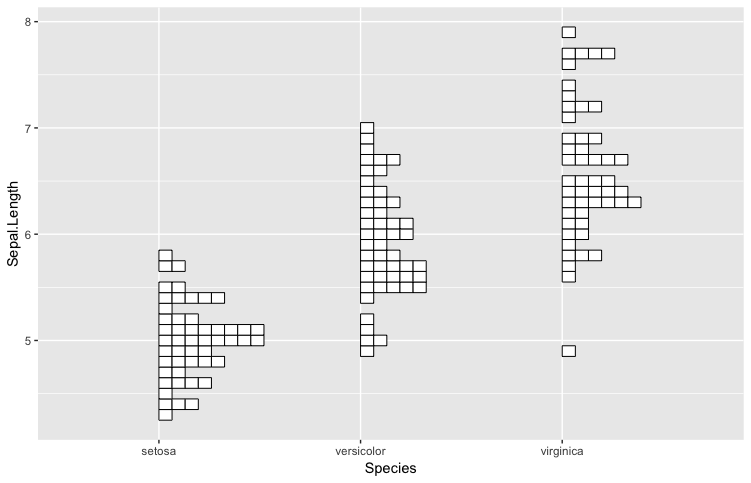The argument binwidth or bins make change bins width.

```ggplot(data = iris) +
geom_brick(aes(y = Sepal.Length, x=Species), binwidth = 0.5)
```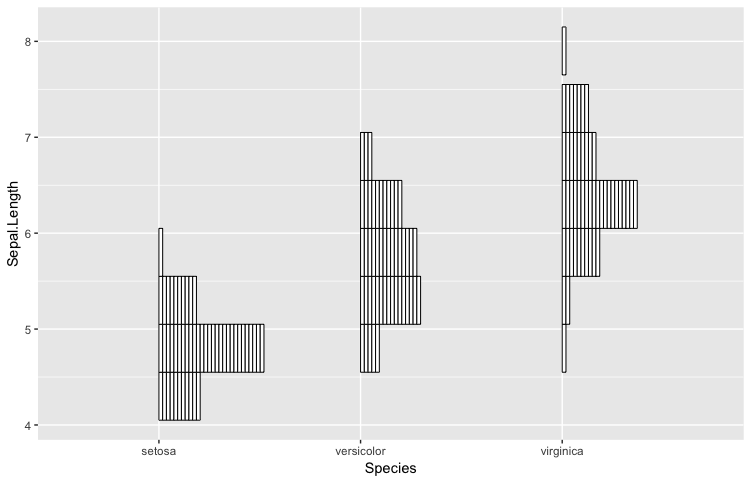fill.

```ggplot(data = iris) +
geom_brick(aes(y = Sepal.Length, x=Species), binwidth = 0.5, fill = "black")
```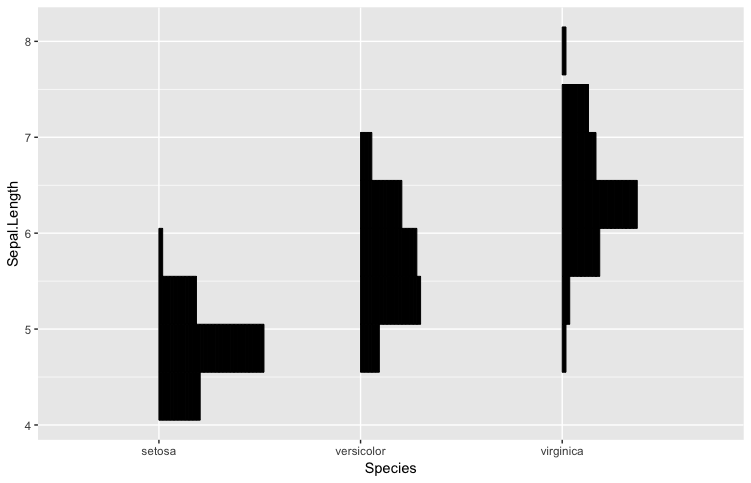You can change the color and stack the rectangles.

```ggplot(data = mpg,aes(y = cty, x=factor(year), fill=factor(cyl))) +
geom_brick(binwidth = 1)
```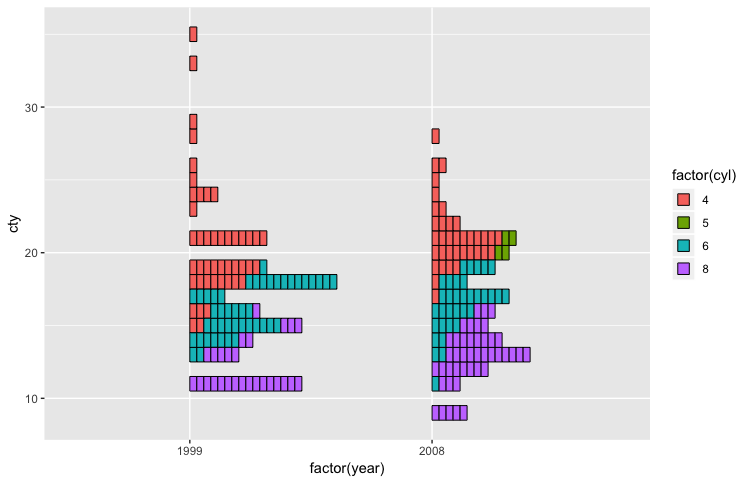If stackgroups = FALSE:

```ggplot(data = mpg,aes(y = cty, x=factor(year), fill=factor(cyl))) +
geom_brick(binwidth = 1, stackgroups = FALSE, alpha = 0.5)
```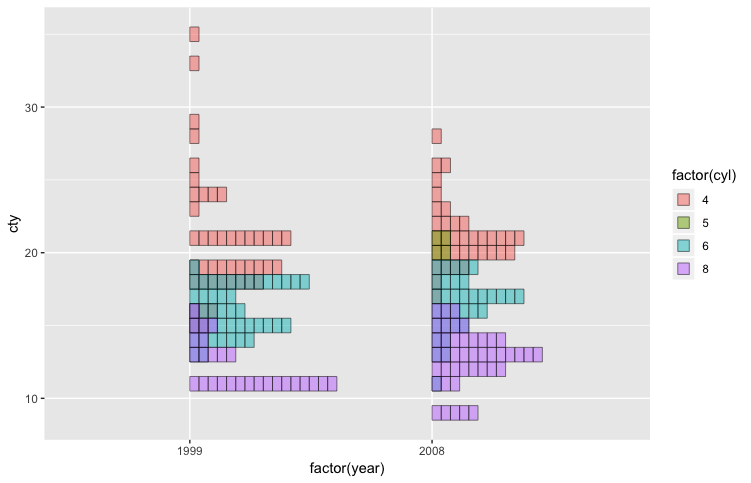If stackdir = "centerwhole":

```ggplot(data = mpg,aes(y = cty, x=factor(year), fill=factor(cyl))) +
geom_brick(binwidth = 1, stackgroups = FALSE, alpha = 0.5,
stackdir = "centerwhole", position = position_dodge(0.5))
```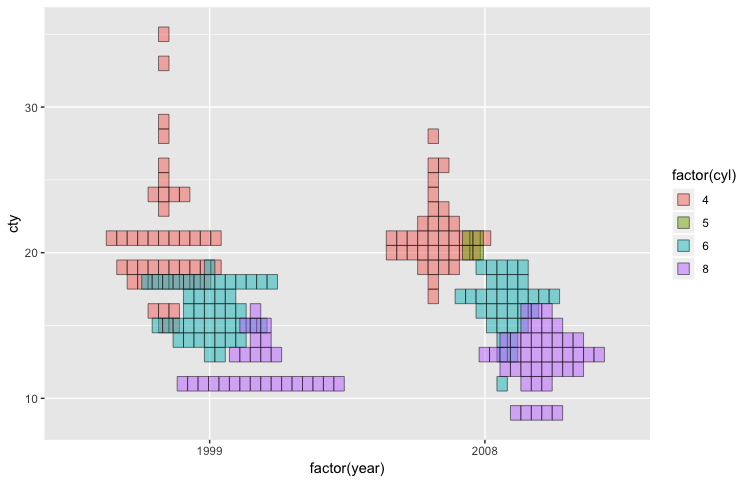When you want to turn sideways, use coord_flip:

```ggplot(data = diamonds, aes(x = color, y=carat, colour=cut)) +
geom_brick(binwidth=0.2) +
coord_flip()
```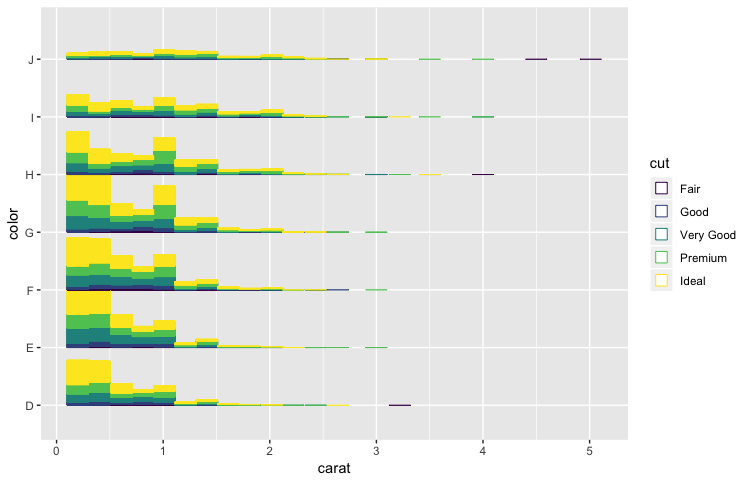```ggplot(data = iris,aes(y = Sepal.Length, x=Species)) +
geom_brick(binwidth = 0.1, stackdir = "centerwhole")+
stat_summary(fun.y = median, fun.ymin = median, fun.ymax = median,
geom = "crossbar")
```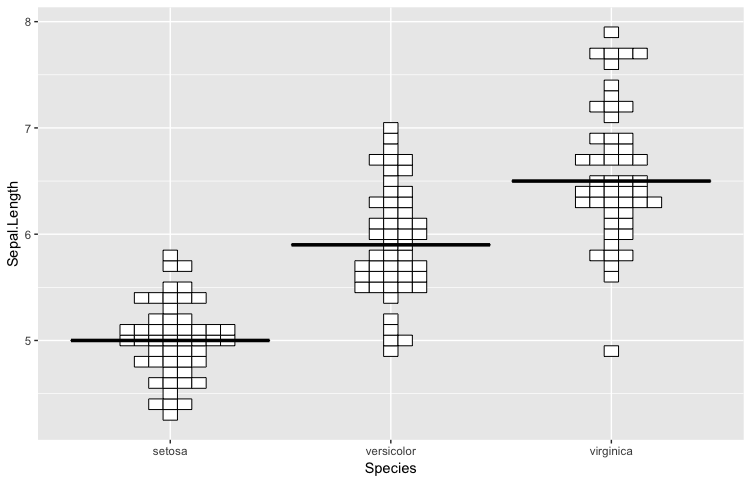You can use facet:

```iris2 <- tidyr::gather(iris,key,value,-Species)
ggplot(data = iris2,aes(y = value, x=Species)) +
geom_brick(binwidth = 0.3,fill="black")+
facet_wrap(~key,scales = "free_y")
```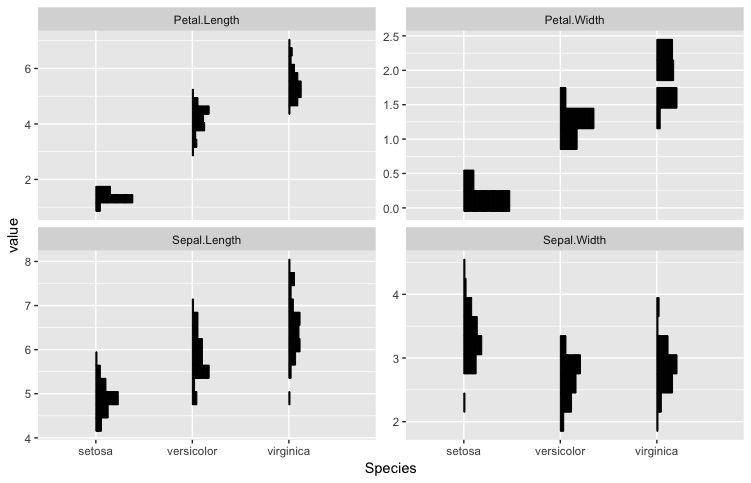### Anscombe's quartet

I'd like to plot the data set available from the following page in several ways.

The Datasaurus Dozen - Same Stats, Different Graphs: Generating Datasets with Varied Appearance and Identical Statistics through Simulated Annealing | Autodesk Research

geom_jitter: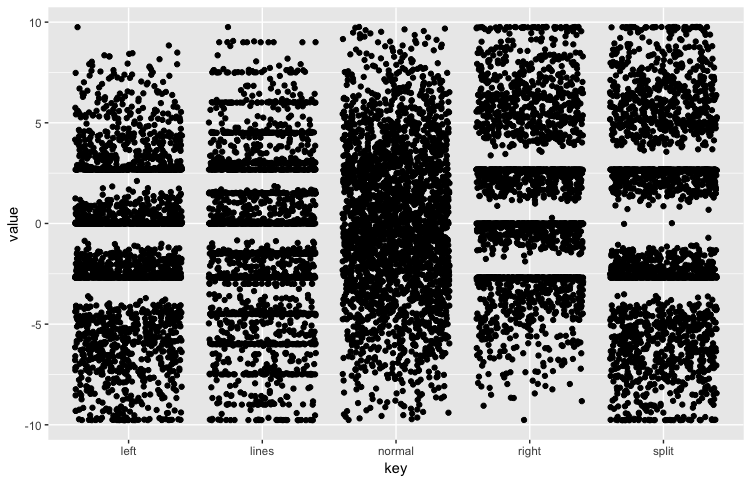It is a visualization which is faithful to the data. However, when the data points increases, it is difficult to show the frequency.

geom_boxplot: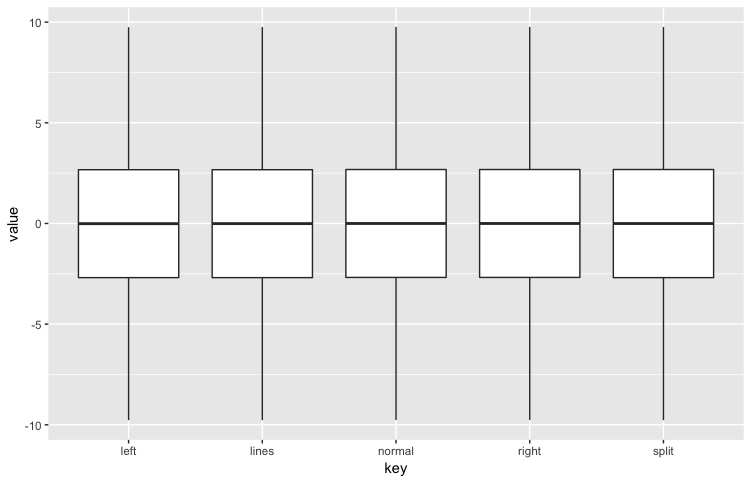The boxplot only shows summarized statistics. In this data set, you can not see any difference in the distributions.

geom_brick: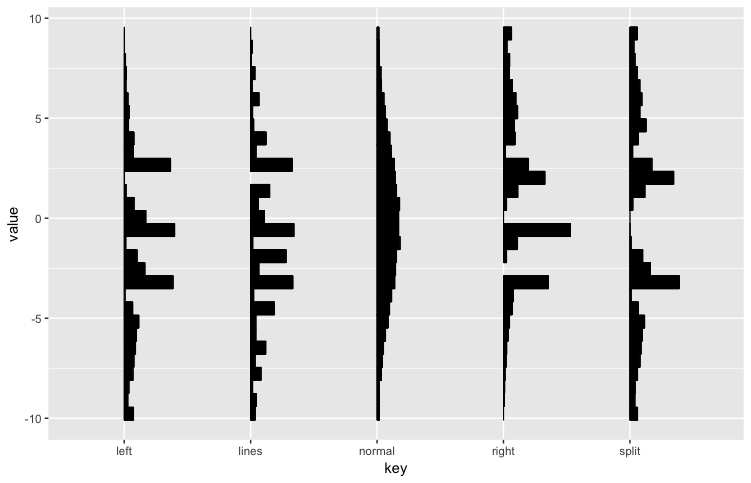I think that the distribution can be understood.

geom_violin: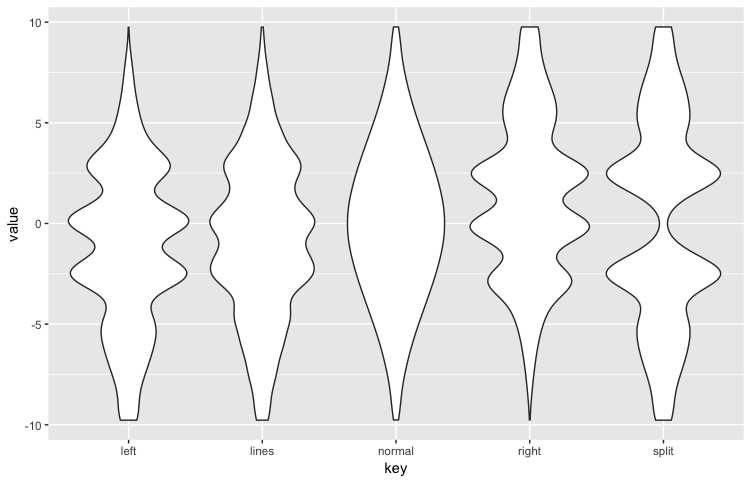pretty good, but the violinplots sometimes make over smoothing.

R code is here:

```library(tidyverse)
library(ggbrick)
dat_t <- gather(dat,key,value,-X1)

ggplot(dat_t,aes(x=key,y=value))+
geom_jitter()

ggplot(dat_t,aes(x=key,y=value))+
geom_boxplot()

ggplot(dat_t,aes(x=key,y=value))+
geom_brick()

ggplot(dat_t,aes(x=key,y=value))+
geom_violin()
```